Courses

# Propagation of Error - Data Analysis, CSIR-NET Physical Sciences Physics Notes | EduRev

## Physics for IIT JAM, UGC - NET, CSIR NET

Created by: Akhilesh Thakur

## Physics : Propagation of Error - Data Analysis, CSIR-NET Physical Sciences Physics Notes | EduRev

The document Propagation of Error - Data Analysis, CSIR-NET Physical Sciences Physics Notes | EduRev is a part of the Physics Course Physics for IIT JAM, UGC - NET, CSIR NET.
All you need of Physics at this link: Physics

The analysis of uncertainties (errors) in measurements and calculations is essential in the physics laboratory.  For example, suppose you measure the length of a long rod by making three measurement x = xbest ± ∆x, y = ybest ± ∆y, and z = zbest ± ∆z.  Each of these measurements has its own uncertainty ∆x, ∆y, and ∆z  respectively.  What is the uncertainty in the length of the rod L = x + y + z?  When we add the measurements do the uncertainties ∆x, ∆y, ∆z cancel, add, or remain the same?  Likewise , suppose we measure the dimensions b = bbest ± ∆b, h = hbest ± ∆h, and w = wbest ± ∆w of a block.  Again, each of these measurements has its own uncertainty ∆b, ∆h, and ∆w  respectively.  What is the uncertainty in the volume of the block V = bhw? Do the uncertainties add, cancel, or remain the same when we calculate the volume?  In order for us to determine what happens to the uncertainty (error) in the length of the rod or volume of the block we must analyze how the error (uncertainty) propagates when we do the calculation.   In error analysis we refer to this as error propagation.

There is an error propagation formula that is used for calculating uncertainties when adding or subtracting measurements with uncertainties and a different error propagation formula for calculating uncertainties when multiplying or dividing measurements with uncertainties.  Let’s first look at the formula for adding or subtracting measurements with uncertainties.

Adding or Subtracting Measurements with Uncertainties.

Suppose you make two measurements,

x = xbest  ±  ∆x
y = ybest  ±  ∆y

What is the uncertainty in the quantity q = x + y or q = x – y?

To obtain the uncertainty we will find the lowest and highest probable value of q = x + y.  Note that we would like to state q in the standard form of q = qbest ± ∆q where

qbest = xbest + ybest

(highest probable value of q = x + y):

(xbest+ ∆x) + (ybest + ∆y) = (xbest+ ybest) + (∆x +∆y) = qbest + ∆q

(lowest probable value of q = x + y):

(xbest- ∆x) + (ybest - ∆y) = (xbest+ ybest) - (∆x +∆y) = qbest – ∆q

Thus, we that

∆q = ∆x + ∆y

is the uncertainty in q = x + y.  A similar result applies if we needed to obtain the uncertainty in the difference q = x – y.  If we had added or subtracted more than two  measurements  x, y, ......, z each with its own uncertainty ∆x, ∆y, ......... , ∆z  respectively , the result would be

∆q = ∆x + ∆y + ......... + ∆z

Now, if the uncertainties ∆x, ∆y, ........., ∆z are random and independent, the result is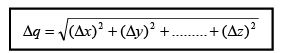Ex. x = 3.52 cm ± 0.05 cm

y = 2.35 cm ± 0.04 cm

Calculate q = x + y  We would like to state q in the standard form of q = qbest ± ∆q

xbest = 3.52cm, ∆x = 0.05cm

ybest = 2.35cm, ∆y = 0.04cm

qbest = xbest + ybest = 3.52cm + 2.35cm = 5.87cm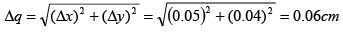q = 5.87cm ± 0.06cm

Multiplying or Dividing Measurements with Uncertainties

Suppose you make two measurements,

x = xbest  ±  ∆x
y = ybest  ±  ∆y

What is the uncertainty in the quantity q = xy or q = x/y?

To obtain the uncertainty we will find the highest and lowest probable value of q = xy.  The result will be the same if we consider q = x/y. Again we would like to state q in the standard form of q = qbest ± ∆q where now qbest = xbest ybest

.(highest probable value of q = xy):

(xbest+ ∆x)(ybest + ∆y) = xbestybest + xbest ∆y +∆x ybest + ∆x ∆y  = qbest + ∆q

= xbestybest + (xbest ∆y + ∆x ybest) =  qbest + ∆q

(lowest probable value of q = xy):

(xbest- ∆x)(ybest - ∆y) = xbestybest - xbest ∆y - ∆x ybest + ∆x ∆y  = qbest – ∆q

= xbestybest – (xbest ∆y + ∆x ybest) =  qbest – ∆q

Since the uncertainties ∆x and ∆y are assumed to be small, then the product ∆x ∆y ≈ 0.  Thus, we see that ∆q = xbest ∆y +∆x ybest in either case.  Dividing by xbestybest gives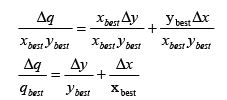Again, a similar result applies if we needed to obtain the uncertainty in the division of     q = x/y.  If we had multiplied or divided more than two measurements  x, y, ......, z each with its own uncertainty ∆x, ∆y, ......... , ∆z  respectively, the result would be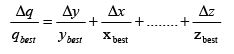Now, if the uncertainties ∆x, ∆y, ........., ∆z are random and independent, the result is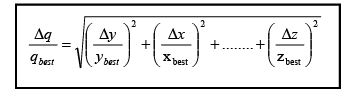Ex.  x = 49.52cm ± 0.08cm
y = 189.53cm ± 0.05cm

Calculate q = xy  We would like to state q in the standard form of q = qbest ± ∆q

xbest = 49.52cm, ∆x = 0.08cm

ybest = 189.53cm, ∆y = 0.05cm

qbest = xbestybest = (49.52cm)(189.53cm)=9.38553 x 103 cm2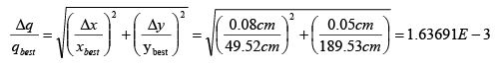∆q = (1.63691 x 10-3)qbest = (1.63691 x 10-3) (9.38553 x 103 cm2)

∆q = 15.3632cm2 ≈ 20 cm

q = 9390 cm2 ± 20 cm2

Uncertainty for a Quantity Raised to a Power

If a measurement x has uncertainty ∆x, then the uncertainty in q = xn, is given by the expression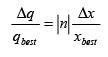Ex.  Let q = x3 where x = 5.75cm ± 0.08 cm.

Calculate the uncertainty ∆q in the quantity q.

We would like to state q in the standard form of q = qbest ± ∆q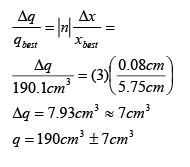159 docs

,

,

,

,

,

,

,

,

,

,

,

,

,

,

,

,

,

,

,

,

,

,

,

,

;